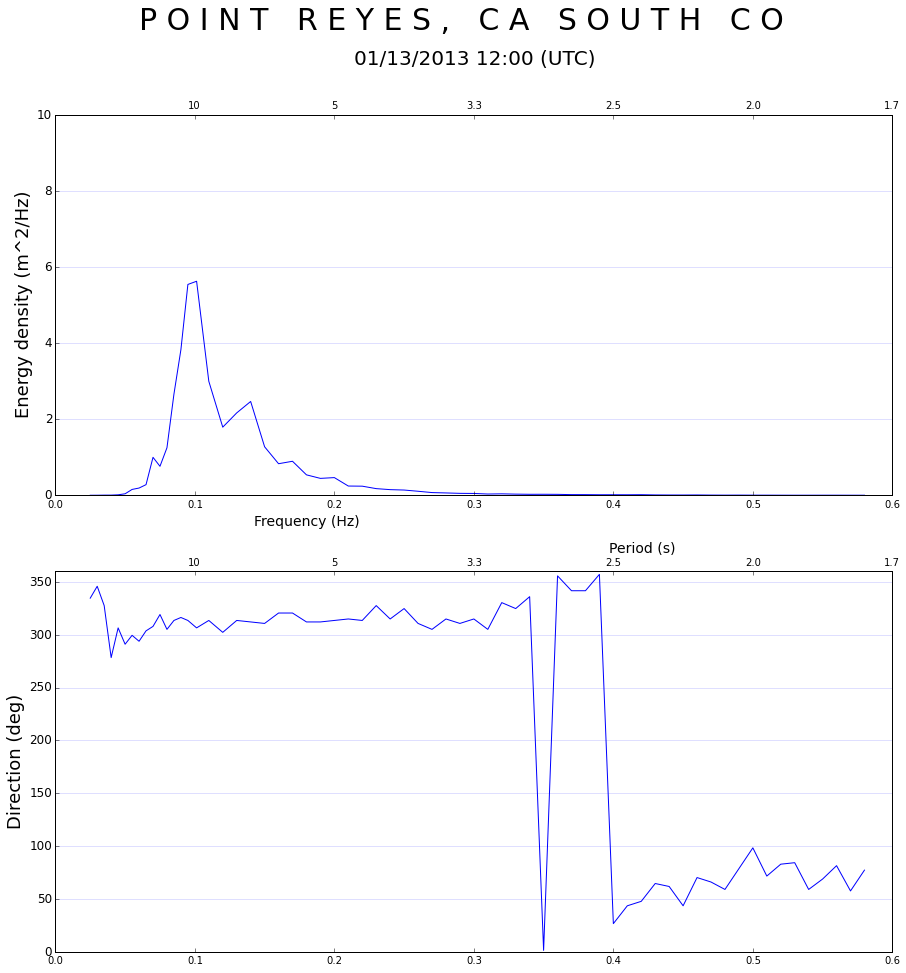# CDIP Station Plot - Spectrum (Ed, Dir)

Create subplots of: Wave Energy Density (Ed) and Direction (Dmean) using OPeNDAP service from CDIP THREDDS Server.

• See http://cdip.ucsd.edu/themes/cdip?pb=1&bl=cdip?pb=1&d2=p1&d2=p70&u3=s:191:st:1:v:spectral_plot for example Spectrum plot.

## Station Number

Enter CDIP Buoy Station Number and year to plot averages

In :
stn = '029'
startdate = "01/13/2013 12:00" # MM/DD/YYYY HH:MM


## Import Libraries

Libraries are Python packages that contain a subset of specific functions

In :
import netCDF4
import numpy as np
import matplotlib.pyplot as plt
import datetime
import time
import calendar


# Data Access

• Data are stored on the CDIP THREDDS server as NetCDF files
• CDIP buoys record data every 30 minutes (48 times/day)

## Import Data as NetCDF from THREDDS URL

CDIP buoy data are available in two subsets:

• Archived data (all past deployments are QCed and aggregated into one NetCDF file for each station)
• Realtime data (current buoy deployment, including present data).

Archived and Realtime NetCDF files must be called using separate URLs

In :
# Comment out the URL that you are not using

# CDIP Archived Dataset URL
data_url = 'http://thredds.cdip.ucsd.edu/thredds/dodsC/cdip/archive/' + stn + 'p1/' + stn + 'p1_historic.nc'

# CDIP Realtime Dataset URL
# data_url = 'http://thredds.cdip.ucsd.edu/thredds/dodsC/cdip/realtime/' + stn + 'p1_rt.nc'


## Open Remote Dataset from CDIP THREDDS Server

In :
nc = netCDF4.Dataset(data_url)


### Read Buoy Variables

Assign variable names to the relevant variables from NetCDF file:

In :
ncTime = nc.variables['waveTime'][:]
Dmean = nc.variables['waveMeanDirection']
Fq = nc.variables['waveFrequency']
Ed = nc.variables['waveEnergyDensity']


Create variables for Buoy Name and Month Name, to use in plot title

In :
buoyname = nc.variables['metaStationName'][:]
buoytitle = " ".join(buoyname[:-40])

month_name = calendar.month_name[int(startdate[0:2])]
year_num = (startdate[6:10])


## Change UNIX timestamps to human dates

NetCDF stores time variables as UNIX dates (values are in seconds since 01-01-1970 00:00:00). The below functions allow us to convert between human-format timestamps (e.g. 'MM/DD/YYYY') and UNIX timestamps.

In :
# Find nearest value in numpy array
def find_nearest(array,value):
idx = (np.abs(array-value)).argmin()
return array[idx]

# Convert to unix timestamp
def getUnixTimestamp(humanTime,dateFormat):
unixTimestamp = int(time.mktime(datetime.datetime.strptime(humanTime, dateFormat).timetuple()))
return unixTimestamp


## Time Index Values

Find the UNIX values that correspond to start (Day 1) and end (Day 28) dates for each month. Then find the array index numbers for each UNIX value within the 'ncTime' arrays.

In :
unixstart = getUnixTimestamp(startdate,"%m/%d/%Y %H:%M")
neareststart = find_nearest(ncTime, unixstart)  # Find the closest unix timestamp
nearIndex = numpy.where(ncTime==neareststart)  # Grab the index number of found date

Index = nearIndex-16 # Subtract 16 index units from the waveTime variable, because it appears to be shifted forward 16 from UTC time.
# NOTE: CDIP plots are displayed in PST (UTC-8 hrs).

In :
print ncTime[nearIndex]
print ncTime[Index]

1358106885
1358078085



## Plot Wave Time-Series

In :
# Create figure and specify figure size
fig = plt.figure(figsize=(15,15))

# Create 2 stacked subplots for Energy Density (Ed) and Mean Direction (Dmean)
pEd = plt.subplot(2,1,1)
pEd.plot(Fq[:],Ed[Index,:],'b')
pDmean = plt.subplot(2,1,2, sharex=pEd)
pDmean.plot(Fq[:],Dmean[Index,:],'b')

# Set titles
plt.suptitle(buoytitle, fontsize=30, y=1)
title(startdate + " (UTC)", fontsize=20, y=2.32)

# Set tick parameters
pEd.tick_params(axis='y', which='major', labelsize=12, right='off')
pDmean.tick_params(axis='y', which='major', labelsize=12, right='off')

# Make secondary x- and y-axes for each graph. Shows both Frequency and Period for x-axes.
pEd2y = pEd.twiny() # Copy x-axis for Graph #1
pDmean2y = pDmean.twiny() # Copy x-axis for Graph #2

# Set axis limits for each plot
# pEdx = pEd.set_xlim(0,0.6)
pEd.set_xlim(0,0.6)
pEd.set_ylim(0,10)
pEd2y.set_xlim(0,0.6)
pDmean.set_ylim(0,360)
pDmean2y.set_xlim(0,0.6)

# Label each axis
pEd.set_xlabel('Frequency (Hz)', fontsize=14, x=0.3)
pEd.set_ylabel('Energy density (m^2/Hz)', fontsize=18)
pDmean.set_ylabel('Direction (deg)', fontsize=18)
pDmean2y.set_xlabel('Period (s)', fontsize=14, x=0.7)

# Format top axis labels to show 'Period' values at tickmarks corresponding to 'Frequency' x-axs
# Top subplot
pEd2y.xaxis.set_major_locator(MaxNLocator(prune='lower'))
pEd2y.set_xticks([0.1,0.2,0.3,0.4,0.5,0.6])
pEd2y.set_xticklabels(['10','5','3.3','2.5','2.0','1.7'])

# Bottom subplot
pDmean2y.xaxis.set_major_locator(MaxNLocator(prune='lower'))
pDmean2y.set_xticks([0.1, 0.2, 0.3,0.4,0.5,0.6])
pDmean2y.set_xticklabels(['10','5','3.3','2.5','2.0','1.7'])

# Plot dashed gridlines
pEd.grid(b=True, which='major', axis = 'y', color='b', alpha = 0.25, linestyle='-')
pDmean.grid(b=True, which='major', axis = 'y', color='b', alpha = 0.25, linestyle='-')